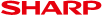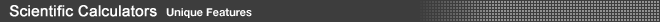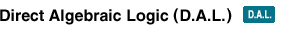Until the introduction of SHARP's D.A.L., keying in equations had been a complicated process making scientific calculators difficult to use. Introduced in 1992 and an industry-first, SHARP's D.A.L. allows symbols and numbers of an equation to be entered as they are written. Instead of wasting energy on difficult calculator operations, users are free to concentrate on mathematical concepts.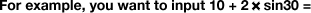• Non D.A.L. system key operation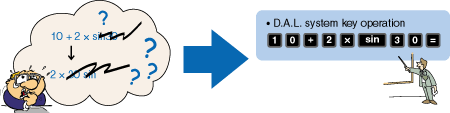You have to push the keys in a different order than the actual equation, making the input process confusing.You push the keys in the same order as the actual equation.